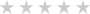Reviews
Target Audience
Course Overview
Course Requirements
Course Syllabus
-
Module 1: Data Analysis in Excel

This module looks at the classic Excel dashboard and at ways to extend it.

Lessons

• Classic Data Analysis with Excel
• Excel Pivot Tables
• Limitations of Classic Data Analysis

Lab : Building a Classic Excel Dashboard

• Formatting Data
• Building a Pivot Table
• Adding pivot charts and a slicer

After completing this module, students will be able to:

• Describe classic data analysis with Excel
• Describe Excel pivot tables
• Describe the limitations of classic data analysis with Excel
-
Module 2: The Excel Data Model

This module looks at the classic Excel data model and at ways to extend it.

Lessons

• Using an Excel Data Model
• DAX

Lab : Explore an Excel Data Model

• Create Calculated Columns
• Format Data Model Data
• Create Measures
• Analyze the Data

After completing this module, students will be able to:

• Describe an Excel data model
• View data within an Excel data table
• Describe DAX
-
Module 3: Importing Data from Files

This module looks at pre-formatting and importing CSV files.

Lessons

• Importing Data into Excel
• Shaping and Transforming Data

Lab : Importing Data from a CSV File

• Import and Transform Data from a CSV File
• Add Data from a Folder

After completing this module, students will be able to:

• Import data into excel.
• Shape and transform data.
-
Module 4: Importing Data from Databases

This module looks at how to import data into Excel from a SQL Server database.

Lessons

• Available Data Sources
• Previewing, Shaping, and Transforming Data
• Table Relationships and Hierarchies

Lab : Import Data from Multiple Sources

• Import Data from SQL Server
• Import Data from a CSV File
• Create a Data Table

After completing this module, students will be able to:

• Identify available data sources.
• Preview, shape, and transform data.
• Explain table relationships and hierarchies.
• Load data from various sources.
-
Module 5: Importing Data from Excel Reports

This module describes how to import data from a report.

Lessons

• Importing Data from Excel Reports
• Transforming Excel report Data

Lab : Importing Data from a Report

• Import Data from Excel
• Transform the Excel Data
• Load the Data into an Excel Data Model

After completing this module, students will be able to:

• Import data from Excel reports.
• Transform Excel report data.
-
Module 6: Creating and Formatting Measures

This module describes how to create and format measures.Lessons

• DAX
• Measures

Lab : Creating Measures using Advanced DAX Functions

• Last year comparison
• Year to date
• Market Share

After completing this module, students will be able to:

• Explain what DAX is and when to use it.
• Describe a measure.
• Use some of the advanced functions within DAX.
-
Module 7: Visualizing Data in Excel

This module describes how to visualize data in Excel.

Lessons

• Pivot Charts
• Cube Functions
• Charts for Cube Functions

Lab : Data Visualization in Excel

• Create a Tabular Report
• Create a Pivot Chart

After completing this module, students will be able to:

• Create and refine a pivot chart.
• Describe cube functions and when to use them.
• Describe a number of charts for use with cube functions.
-
Module 8: Using Excel with Power BI

This module describes how to use Excel with Power BI.

Lessons

• Power BI
• Power BI Mobile App

Lab : Creating a Power BI Dashboard with Excel